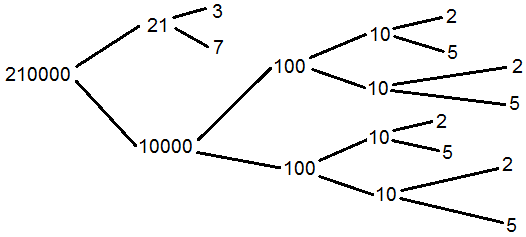# How to Find the Prime Factorization of a Number with a Factor Tree

Prime Factorization Calculator

Enter a positive integer with at most 15 digits below:

A prime number is an integer that can be divided only by 1 and itself. (0 and 1 are not considered prime.) Every positive integer greater than 1 can be broken down into the product of prime factors. For example, the prime factorization of 300 is 2·2·3·5·5. If the number itself is prime, then of course it has only one prime factor--itself!

You can find the prime decomposition of a number by creating a factor tree that branches out into smaller and smaller factors. At the end of the process you will be left with only the prime factors at the ends of the branches.

Apply the steps below to find the prime decomposition by hand, or use the calculator on the left.

(Step 1) As an example to illustrate the steps, let's create a factor tree for 210000. The first step is to break the number into the product of two factors, not necessarily prime.

There are usually several ways to do this, and it doesn't matter how you start the process since all branchings eventually lead to the same prime factorization at the end. For the number 210000, we could split it into either 700 times 300, or 21 times 10000. Let's take the latter factorization.

(Step 2) Take the two factors you found in Step 1 and factor each one. For example, 21 splits into 3 and 7, while 10000 splits into 100 and 100. Since 3 and 7 are already prime, we can stop working on that branch.

(Step 3) Continue factoring each branch until you arrive at only prime factors. For example, each 100 splits into 10 and 10, and each 10 further splits into 2 and 5. See diagram below:(Step 4) Record all of the numbers at the ends of the branches to obtain the prime factorization. As you can see from above, the prime factorization of 210000 is 2·2·2·2·3·5·5·5·5·7.